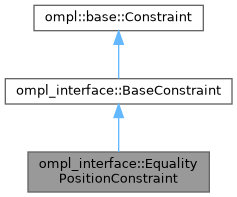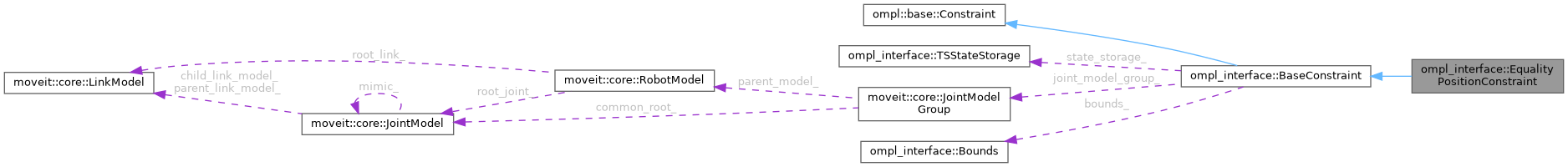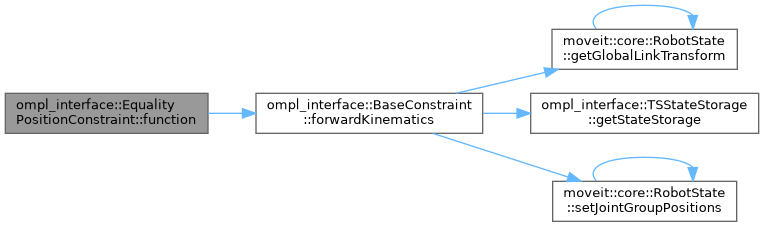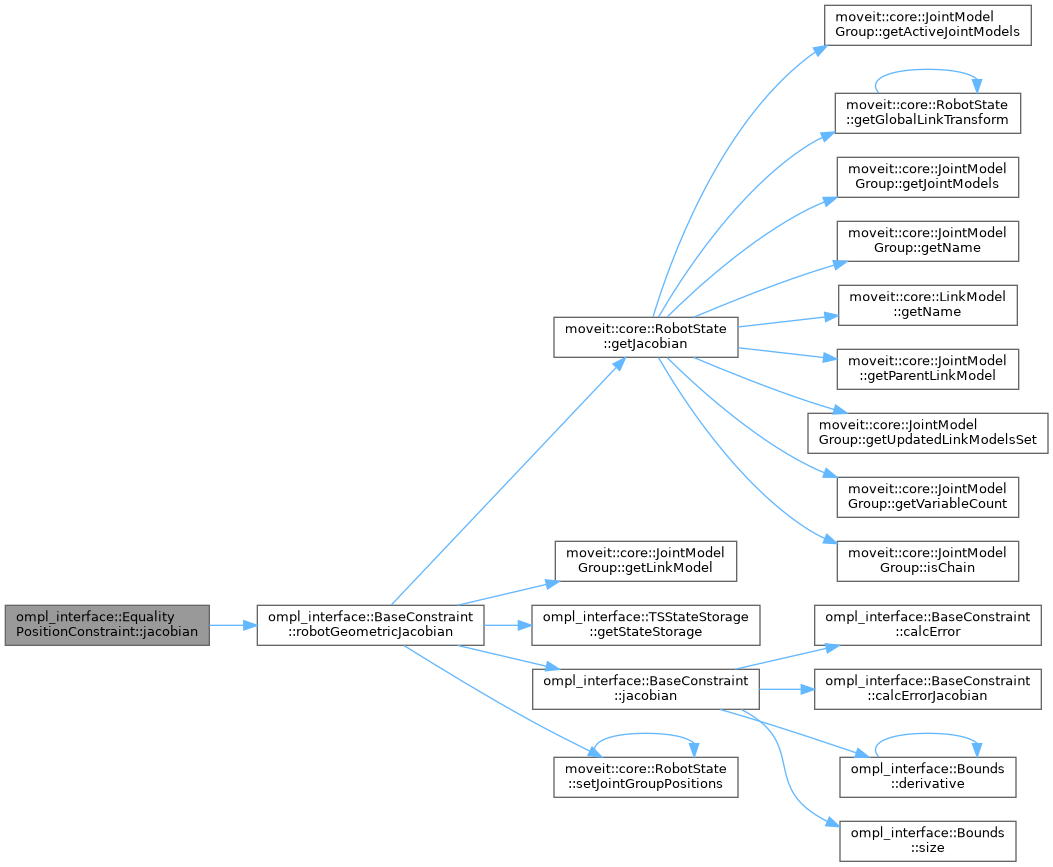moveit2 The MoveIt Motion Planning Framework for ROS 2.
ompl_interface::EqualityPositionConstraint Class Reference

Equality constraints on a link's position. More...

`#include <ompl_constraints.h>`

Inheritance diagram for ompl_interface::EqualityPositionConstraint:[legend]
Collaboration diagram for ompl_interface::EqualityPositionConstraint:[legend]

## Public Member Functions

EqualityPositionConstraint (const moveit::core::RobotModelConstPtr &robot_model, const std::string &group, const unsigned int num_dofs)

void parseConstraintMsg (const moveit_msgs::msg::Constraints &constraints) override
Parse bounds on position parameters from MoveIt's constraint message. More...

void function (const Eigen::Ref< const Eigen::VectorXd > &joint_values, Eigen::Ref< Eigen::VectorXd > out) const override

void jacobian (const Eigen::Ref< const Eigen::VectorXd > &joint_values, Eigen::Ref< Eigen::MatrixXd > out) const overridePublic Member Functions inherited from ompl_interface::BaseConstraint
BaseConstraint (const moveit::core::RobotModelConstPtr &robot_model, const std::string &group, const unsigned int num_dofs, const unsigned int num_cons=3)
Construct a BaseConstraint using 3 `num_cons` by default because all constraints currently implemented have 3 constraint equations. More...

void init (const moveit_msgs::msg::Constraints &constraints)
Initialize constraint based on message content. More...

void function (const Eigen::Ref< const Eigen::VectorXd > &joint_values, Eigen::Ref< Eigen::VectorXd > out) const override

void jacobian (const Eigen::Ref< const Eigen::VectorXd > &joint_values, Eigen::Ref< Eigen::MatrixXd > out) const override
Jacobian of the constraint function. More...

Eigen::Isometry3d forwardKinematics (const Eigen::Ref< const Eigen::VectorXd > &joint_values) const
Wrapper for forward kinematics calculated by MoveIt's Robot State. More...

Eigen::MatrixXd robotGeometricJacobian (const Eigen::Ref< const Eigen::VectorXd > &joint_values) const
Calculate the robot's geometric Jacobian using MoveIt's Robot State. More...

virtual Eigen::VectorXd calcError (const Eigen::Ref< const Eigen::VectorXd > &) const
For inequality constraints: calculate the value of the parameter that is being constrained by the bounds. More...

virtual Eigen::MatrixXd calcErrorJacobian (const Eigen::Ref< const Eigen::VectorXd > &) const
For inequality constraints: calculate the Jacobian for the current parameters that are being constrained.

• This error jacobian, as the name suggests, is only the jacobian of the position / orientation / ... error. It does not take into account the derivative of the penalty functions defined in the Bounds class. This correction is added in the implementation of of BaseConstraint::jacobian.
More...

const Eigen::Vector3d getTargetPosition ()

const Eigen::Quaterniond getTargetOrientation ()Protected Attributes inherited from ompl_interface::BaseConstraint
TSStateStorage state_storage_
Thread-safe storage of the robot state. More...

const moveit::core::JointModelGroupjoint_model_group_

Robot link the constraints are applied to. More...

Bounds bounds_
Upper and lower bounds on constrained variables. More...

Eigen::Vector3d target_position_
target for equality constraints, nominal value for inequality constraints. More...

Eigen::Quaterniond target_orientation_
target for equality constraints, nominal value for inequality constraints. More...

## Detailed Description

Equality constraints on a link's position.

When you set the name of a constraint to 'use_equality_constraints', all constraints with a dimension lower than `EQUALITY_CONSTRAINT_THRESHOLD` will be modelled as equality constraints.

The dimension value for the others are ignored. For example, a box with dimensions [1.0, 1e-5, 1.0] will result in equality constraints on the y-position, and no constraints on the x or z-position.

TODO(jeroendm) We could make this a base class `EqualityConstraints` with a specialization for position and orientation constraints in the future. But the direct overriding of `function` and `jacobian` is probably more performant.

Definition at line 309 of file ompl_constraints.h.

## ◆ EqualityPositionConstraint()

 ompl_interface::EqualityPositionConstraint::EqualityPositionConstraint ( const moveit::core::RobotModelConstPtr & robot_model, const std::string & group, const unsigned int num_dofs )

Definition at line 227 of file ompl_constraints.cpp.

## ◆ function()

 void ompl_interface::EqualityPositionConstraint::function ( const Eigen::Ref< const Eigen::VectorXd > & joint_values, Eigen::Ref< Eigen::VectorXd > out ) const
override

Definition at line 268 of file ompl_constraints.cpp.

Here is the call graph for this function:## ◆ jacobian()

 void ompl_interface::EqualityPositionConstraint::jacobian ( const Eigen::Ref< const Eigen::VectorXd > & joint_values, Eigen::Ref< Eigen::MatrixXd > out ) const
override

Definition at line 286 of file ompl_constraints.cpp.

Here is the call graph for this function:## ◆ parseConstraintMsg()

 void ompl_interface::EqualityPositionConstraint::parseConstraintMsg ( const moveit_msgs::msg::Constraints & constraints )
overridevirtual

Parse bounds on position parameters from MoveIt's constraint message.

This can be non-trivial given the often complex structure of these messages.

Implements ompl_interface::BaseConstraint.

Definition at line 233 of file ompl_constraints.cpp.

The documentation for this class was generated from the following files: